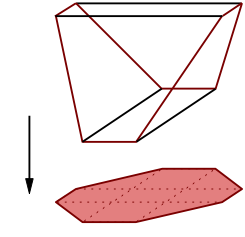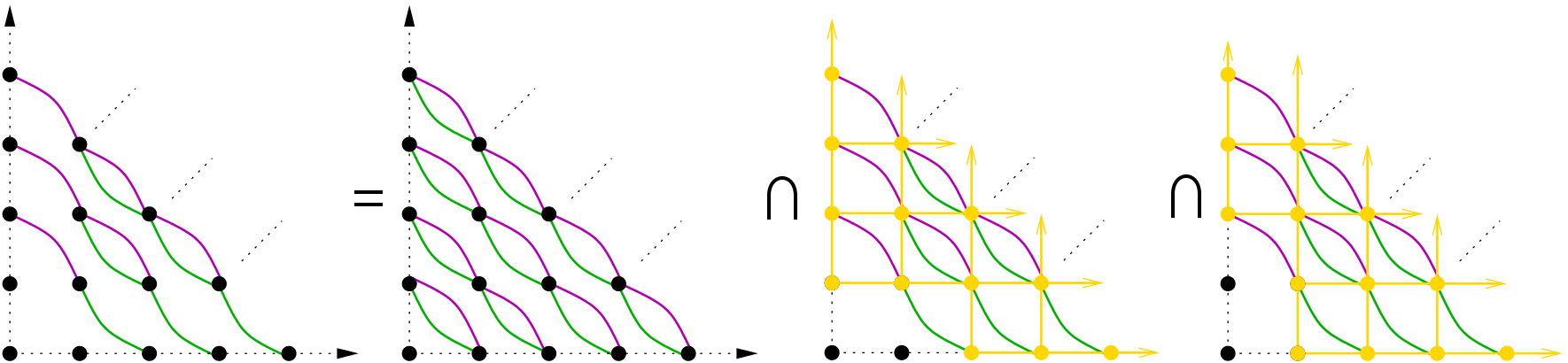×
Complexity Reduction

We understand complexity as an intrinsic property that makes it difficult to determine an appropriate mathematical representation of a real world problem, to assess the fundamental structures and properties of mathematical objects, or to algorithmically solve a given mathematical problem. By complexity reduction we refer to all approaches that help to overcome these difficulties in a systematic way and to achieve the aforementioned goals more efficiently.

For many mathematical tasks, approximation and dimension reduction are the most important tools to obtain a simpler representation and computational speedups. We see complexity reduction in a more general way and will also, e.g., investigate liftings to higher-dimensional spaces and consider the costs of data observation.

##### Example: Lifting of Polytopes into Higher Dimensional SpacesThe complexity of the descriptions of polytopes can be significantly reduced by representing them as projections of higher dimensional ones. In this example, we represent a two-dimensional polytope that requires eight inequalities by a three dimensional polytope that can be described by only six inequalities. In higher dimensions the effect can be far more drastic.

##### Example: Solving binomial equations combinatoriallyMesoprimary decomposition helps to solve complex systems of non-linear equations by means of simple combinatorial methods. The solutions of non-linear equations often vary dramatically depending on whether one searches for them in the real or the complex numbers. Mesoprimary decomposition allows to go quite far in the solution process without this distinction, which then only appears in a very simple subroutine in the end of the process. For example, finding the solutions of the equations x2=xy and xy=y2 becomes just a simple computation with pictures as above.

##### Example: Dimension Reduction for Dynamical Systems
Dimension reduction for dynamical systems is a powerful methodology that allows analysis and control of large scale and spatially distributed systems. Basic principles and underlying concepts like proper orthogonal decomposition or reduced basis methods will be useful to almost everyone working in complexity reduction. The problem of efficiently choosing the number and the timing of snapshots in these methods is very similar to the corresponding task in optimal experimental design (OED), i.e., to determine data samplings that maximize information concerning model structure or model parameters. We expect a high potential for approaches from OED in model reduction.

##### Example: Lifting of Optimization and Control Problems
Lifting of optimization and control problems to higher dimensional spaces may reduce the number of algorithmic iterations, as in the case of the Lifted Newton method, or the amount of enumerative work done by Branch-and-Lift-and-Project algorithms. It may also lead to a more concise (e.g., polynomial instead of exponential) representation of the underlying feasible region by means of extended formulations via polyhedral or more general semialgebraic sets.

...more